# Exercise 7.2.16 Use the dimension theorem to prove Theorem 1.3.1: If A is an m x...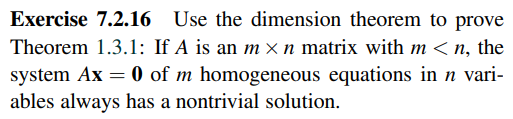Exercise 7.2.16 Use the dimension theorem to prove Theorem 1.3.1: If A is an m x n matrix with m <n, the system Ax = 0 of m homogeneous equations in n vari- ables always has a nontrivial solution.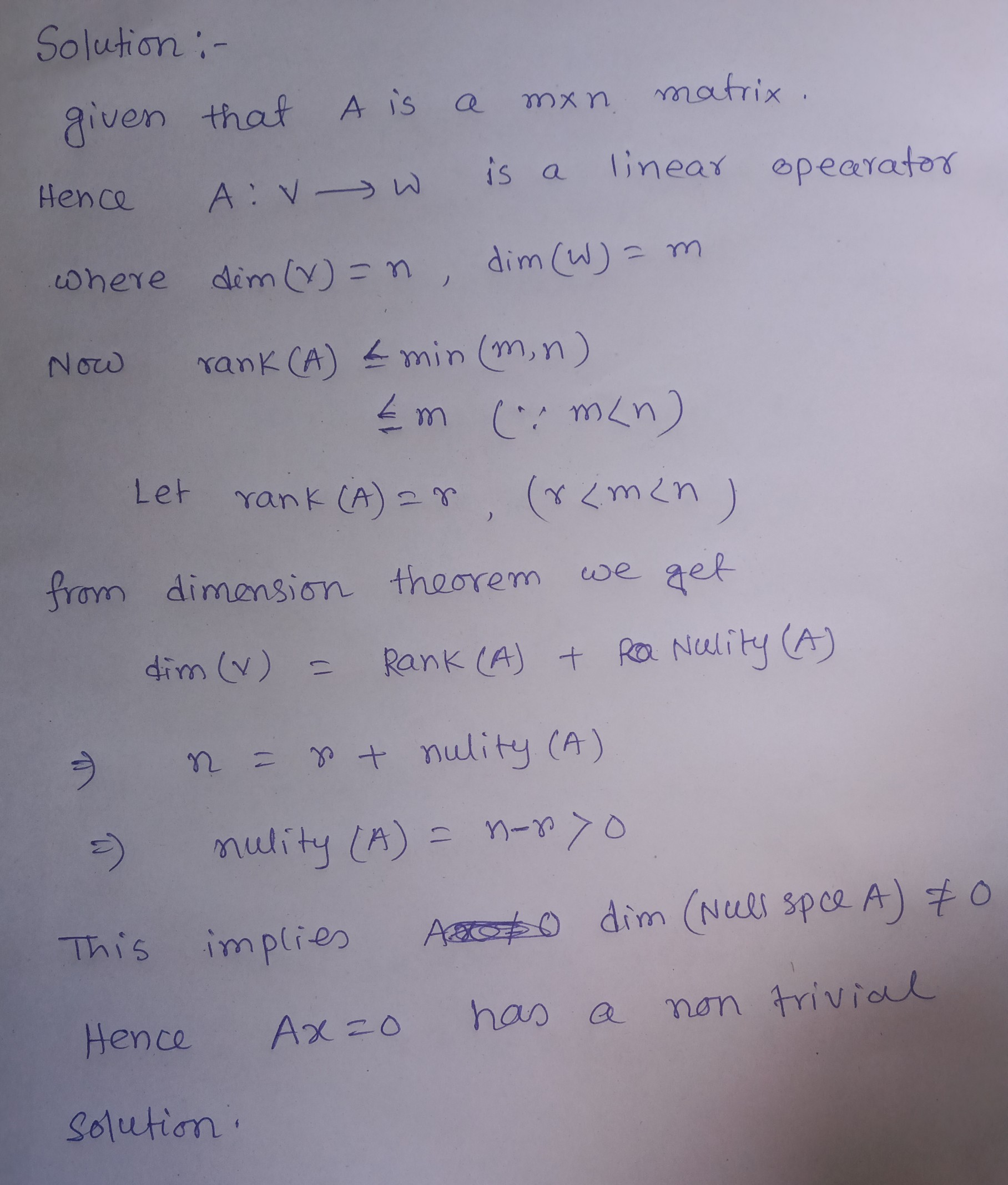##### Add Answer of: Exercise 7.2.16 Use the dimension theorem to prove Theorem 1.3.1: If A is an m x...
Similar Homework Help Questions
• ### 5. Use Rice's Theorem to prove the undecidablity of the following language. P = {< M...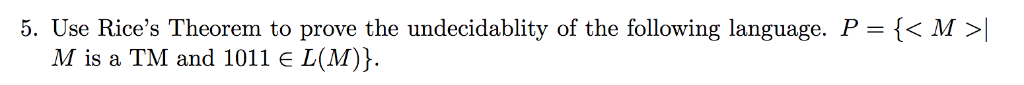5. Use Rice's Theorem to prove the undecidablity of the following language. P = {< M > M is a TM and 1011 E L(M)}.

• ### Use the properties of a Cauchy-Euler system to find a general solution of the given system....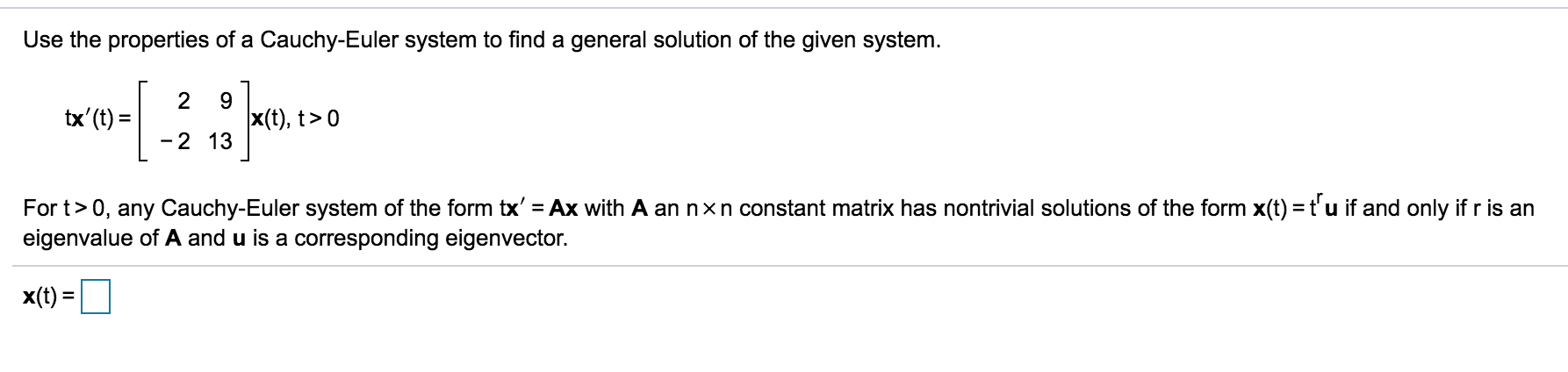Use the properties of a Cauchy-Euler system to find a general solution of the given system. 2 9 tx'(t) X(t), t> 0 -2 13 For t>0, any Cauchy-Euler system of the form tx' = Ax with A an nxn constant matrix has nontrivial solutions of the form x(t) = t’u if and only if ris an eigenvalue of A and u is a corresponding eigenvector. x(t) =

• ### Use the properties of a Cauchy-Euler system to find a general solution of the given system....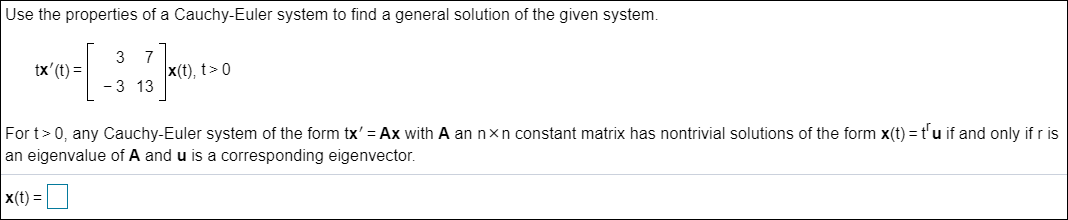Use the properties of a Cauchy-Euler system to find a general solution of the given system. 3 7 tx'(t)= X(t), t> - 3 13 For t>0, any Cauchy-Euler system of the form tx' = Ax with A an nxn constant matrix has nontrivial solutions of the form x(t)= t'u if and only ifr is an eigenvalue of A and u is a corresponding eigenvector. x(t) = 0

• ### Use induction and Pascal's identity to prove that (7) = 2" where n > 0.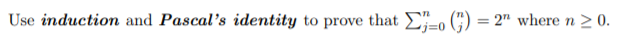Use induction and Pascal's identity to prove that (7) = 2" where n > 0.

• ### Use the properties of a Cauchy-Euler system to find a general solution of the given system....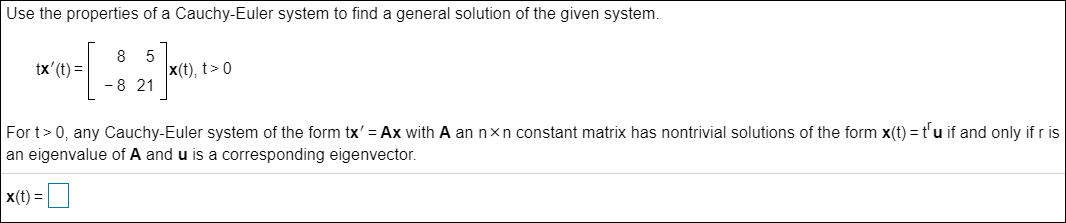Use the properties of a Cauchy-Euler system to find a general solution of the given system. 8 5 tx' (t) = X(t), t> 0 - 8 21 For t>0, any Cauchy-Euler system of the form tx' = Ax with A an nxn constant matrix has nontrivial solutions of the form x(t) = t'u if and only if ris an eigenvalue of A and u is a corresponding eigenvector. X(t) = 0

• ### Use the properties of a Cauchy-Euler system to find a general solution of the given system....Use the properties of a Cauchy-Euler system to find a general solution of the given system. 8 5 tx' (t) = X(t), t> 0 - 8 21 For t>0, any Cauchy-Euler system of the form tx' = Ax with A an nxn constant matrix has nontrivial solutions of the form x(t) = t'u if and only if ris an eigenvalue of A and u is a corresponding eigenvector. X(t) = 0

• ### Now assume that f(0) = 0 and f'(0) = 0. Prove that if f is twice...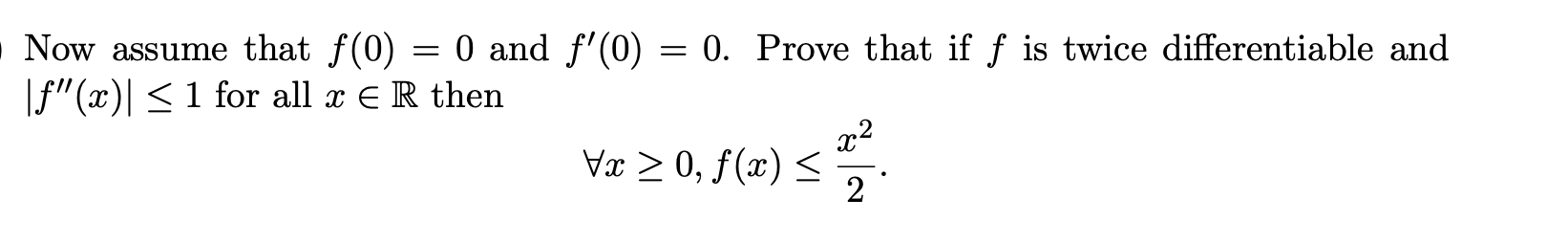Now assume that f(0) = 0 and f'(0) = 0. Prove that if f is twice differentiable and If"(x) < 1 for all x E R then 22 Vx > 0, f(x) < 2

• ### Use induction to prove that 0–0 4j3 = n4 + 2n3 + n2 where n >...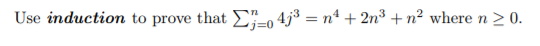Use induction to prove that 0–0 4j3 = n4 + 2n3 + n2 where n > 0.

• ### (7) Green's Theorem for Work in the Plane F(x, y) =< M, N >=< x, y2...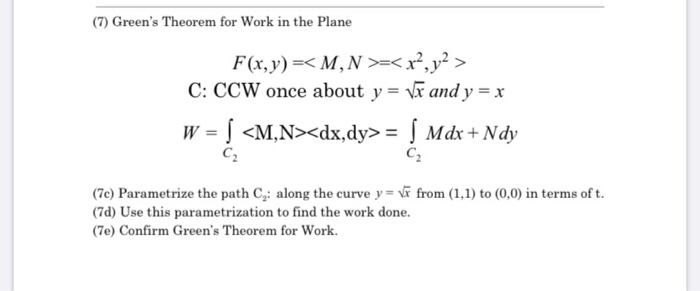(7) Green's Theorem for Work in the Plane F(x, y) =< M, N >=< x, y2 > C: CCW once about y = vw and y = x W = | <M,N><dx,dy>= | Mdx + Ndy CZ CZ (70) Parametrize the path Cy: along the curve y = vw from (1,1) to (0,0) in terms of t. (70) Use this parametrization to find the work done. (7e) Confirm Green's Theorem for Work. (7) Green's Theorem for Work in the Plane...

• ### Let F = < x-eyz, xexx, z?exy >. Use Stokes' Theorem to evaluate slice curlĒ ds,...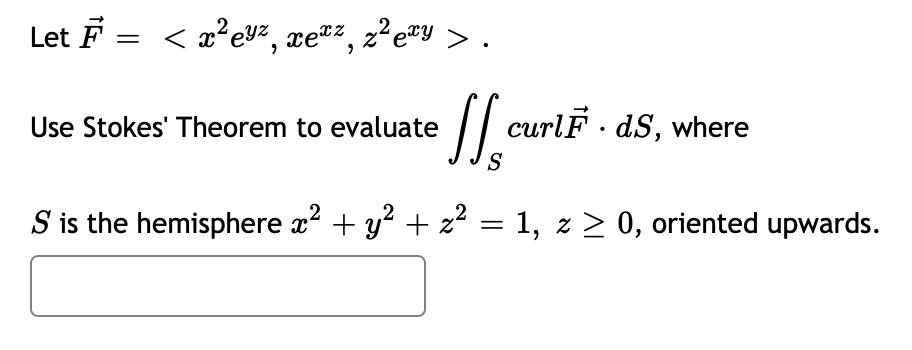Let F = < x-eyz, xexx, z?exy >. Use Stokes' Theorem to evaluate slice curlĒ ds, where S is the hemisphere x2 + y2 + z2 = 1, 2 > 0, oriented upwards.

Free Homework App